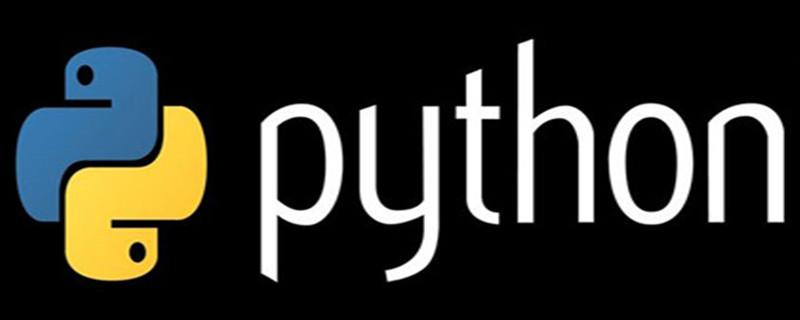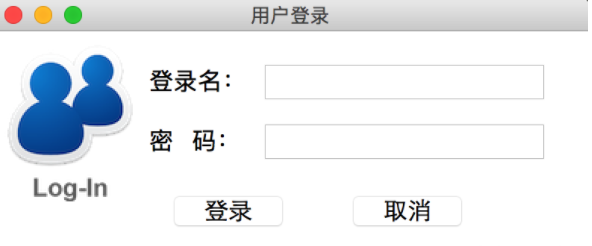• 写文章
• 提问

# python tkinter中place绝对布局怎么用？place用法：

`place(x=10,y=20)`

Place介绍：

place布局是直接定义绝对位置的，但是定义时候必须要传入参数才可以，像上面介绍的用法一样，按照那种模式执行即可。

```from tkinter import *
root = Tk()
root.title("用户登录")
root.geometry("400x180")
Label_username = Label(root,text = "登录名："place(x = 50,y = 20)
Entry_username = Entry(root,font = "place(x = 120,y = 20)
Label_password = Label(root,text = "密   码："place(x = 50,y = 60)
Entry_password = Entry(root,font = place(x = 120,y = 60)
Button_login = Button(root,text = "登录",font = place(x = 70,y = 120)
Button_cancer = Button(root,text = "取消",font =place(x = 210,y = 120)
root.mainloop()```